e-ISSN: 2319-9849

All submissions of the EM system will be redirected to Online Manuscript Submission System. Authors are requested to submit articles directly to Online Manuscript Submission System of respective journal.

# Thermodynamics of the Solvation of Lead Nitrate in Mixed Acetone-H2O Solvents at Different Temperatures

Esam A Gomaa1*, Elsayed M Abou Elleef2, Ahmed Fekri1, Mohamed Khairy3 and Reham M Abou Karn1

1Chemistry Department, Faculty of Science, Mansoura University, 35516-Mansoura, Egypt

2Basic Science Departments, Delta Higher Institute for Engineering & Technology, 35681- Dakhlia, Mansoura, Egypt

3Chemistry Department,Faculty of Science, Benha University, Benha, Egypt

*Corresponding Author:
Esam A Gomaa
Chemistry Department, Faculty of Science
Mansoura University, 35516-Mansoura, Egypt

Received date: 18/05/2014; Revised date: 20/06/2014; Accepted: 21/06/2014

Visit for more related articles at Research & Reviews: Journal of Chemistry

## Abstract

The Gibbs free energies as very important thermodynamic property were evaluated for Pb(NO3)2 in mixed Acetone-H2O solvents at different temperature from the experimental solubility measurements. The ratio of the ionic between lead and nitrate ions was used to divide the total Gibbs free energy of the salt into its individual contribution in the mixtures used. The conventional Gibbs free energies for the cation (Pb2+) and anion (NO- 3) were estimated theoretically and also the Gibbs free energy of NO- 3 gas was evaluated and their values were discussed.

#### Keywords

Pb(NO3)2; Gibbs free energy of salvation; Lead nitrate; aqueous- acetone mixtures; conventional free energies.

#### Introduction

For neutral species Experimental solvation Gibbs free energies have been tabulated large number of solutes in both aqueous [1-7] and non-aqueous [7,8] solvents. Typically, these solvation free energies are determined Experimentally  and their uncentainity is relatively low ( ~ 0.8 KJ/mol) .

Determining accurate values for the Gibbs free energies of ionic solutes like Pb(NO3)2 is important than that of neutral solutes understanding the partitioning of single ions between different liquid phases is important in many areas of biology. For example, the electrical singles sent by nerve cells are activated by changes in cell potential that are caused by the movement of various ions across the neuronal membrane . The division of thermodynamic Gibbs free energies of solvation of electrolytes into ionic constituents is conventionally accomplished by using the single ion solvation Gibbs free energy of one reference ion, conventionally, the proton, to set the single ion scales [11,12]. The aim of this work is to estimate the single ion Gibbs free energies for Pb2+ & NO-3 ions in mixed acetone (Ac)-H2O solvents at different temperatures. A number of different extra thermodynamic approximations have been used [13-25] to partition the sums of cation and anion Gibbs free energies into single ion contribution.

Relative and conventional solvation free energies of ions

The Gibbs solvation free energies of ions are often tabulated as relative free energies by setting the free energy of polvaiton of some reference ion equal zero . Proton was chosen as reference ion. For ions, this result in a set of convertional free energies of solvation that the cations are shifted from their absolute values by the value for the absolute Gibbs solvation free energy of the proton.

The conventional Gibbs free energies of solvation for anions are shifted by an equal amount in the opposite direction. Conventional Gibbs free energies from reduction potentials:

When the convention for the absolute Gibbs free energy of the proton is followed, the solution phase free energy change associated with the half cell for reaction of hydrogen gas is equal to zero. Reduction potentials following this convention for hydrogen electrode are referred as standard reduction potentials. Form the half cell reaction for the reduction of metal cation to crystalline phase and the half reduction reaction of hydrogen gas, the redox reaction can be illustrated through the use of thermochemical cycle . This last procedure can be used to estimate the gas free energy of formation of NO-3 ion, to explain the ionic behaviour.

Experimental

Lead nitrate Pb(NO3)2 GCC-laboratory reagert and n-propanol from Merck Co. were used.

Saturated solutions of Pb(NO3)2 were prepared by dissolving different amounts in closed test tubes containing different Acetone-H2O mixtures. These mixtures were then saturated with nitrogen gas an inert atmosphere. The tubes were placed in a shaking thermostat (Model Gel) for a period of four days till equilibrium reached. The solubility of Pb(NO3)2 in each mixture was measured gravimetrically by evaporating 1 ml of the saturated solution in smallbeaker using I. R. lamp. The measurements were done by three readings for each solutionat 293.15 K, 298.15K,303.15K and 308.15 K.

#### Results and Discussion

The molar solubility (S) for Pb(NO3)2 at 293.15 K,298.15 K,303.15 K and 308.15 K were measured gravimetrically with average of the second number after comma in water, acetone and their mixtures. The solubility values for Pb(NO3)2 are cited in Table 1,Table 2, Table 3 and Table 4. The mean activity coefficient (log γ ± ) of ions which can be estimated from the Debye-Hückel limiting law, asmodified by Robinson and Stokes [28,29-80]].

Table 1: Solubility and Gibbs free energies for Pb(NO3)2 in mixed acetone-H2O solvents at 293.15 K.

Table 2: Solubility and Gibbs free energies for Pb(NO3)2 in mixed acetone-H2O solvents at 298.15 K.

Table 3: Solubility and Gibbs free energies for Pb(NO3)2 in mixed acetone-H2O solvents at 303.15 K.

Table 4: Solubility and Gibbs free energies for Pb(NO3)2 in mixed acetone-H2O solvents at 308.15 K.

Table 5: Single ion Gibbs free energies for Pb2+ and nitrate and their half conventional free energies at 298.15 K. in mixed acetone-H2O solvents (in kJmol-1)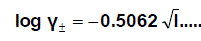(1)

Where I is the ionic strength calculated from (s) values these data ( log γ± ) were tabulated also in Table (1). The solubility product was calculated by the use of equation 2 [30-99].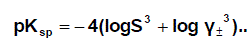(2)

The solbility product (pKsp) data are given in Table (1) from solubility products the Gibbs free energies of solvation and the transfer Gibbs free energies from water to mixed solvents were calculated by using equations (3) and (4).

Their values are tabulated also in Table (1) [31,32].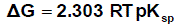(3)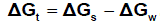(4)

(s), (w) denote solvent and water, respectively.

It was concluded that the Gibbs free energies of transfer (ΔGt ) increase in negativity byincreasing the mole fraction of acetone in the mixed acetone-H2O solvents indicating thespontaneous nature of Pb(NO3)2 solubilization. This is due to more solvation behaviour inthe mixed solvents than that of water where the Gibbs free energy values provideinformation on whether the process conditions favor or disfavor Pb(NO3)2 solubilization in the aqueous carrier solution. Negative Gibbs free energy values indicate favorable conditions. (Fig. 1 - 4)

Single ion Gibbs free energies and conventional free energies for Pb2+ and NO3- ions

Figure 1: Gibbs free energies of transfer (ΔGt) for Pb(NO3)2 versus the mole fraction (Xs) of acetone at 293.15 K

Figure 2: Gibbs free energies of transfer (ΔGt) for Pb(NO3)2 versus the mole fraction (Xs) of acetone at 298.15 K

Figure 3: Gibbs free energies of transfer (ΔGt) for Pb(NO3)2 versus the mole fraction (Xs) of Acetone at 303.15 K

Figure 4: Gibbs free energies of transfer (ΔGt) for Pb(NO3)2 versus the mole fraction (Xs) of acetone at 308.15 K

It was well known that the preferrentional single ion thermodynamic parameters depend on the ionic radii of two ions (cation and anion). Therefore the ionic radii ratio between Pb2+ and NO3- were evaluated from exact radii values given in literature  and found to be 0.1304. Multiply this ratio by the Gibbs free energies of Pb(NO3)2 we get the ionic Gibbs free energies of Pb2+ ion. This last value was substracted from the Pb(NO3)2. Gibbs free energy we obtain the Gibbs free energy for NO3- anion in Pb(NO3)2. the obtained values for single ions are presented in Table (2). The conventional Gibbs free energies ΔG s*con (Pb2+) for Pb2+ ion in solvents are shifted from their absolute values by the absolute free energy of the proton  according to equation (5)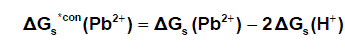(5)

and for NO3- anion is shifted by an equal amount in the opposite direction (equation 6).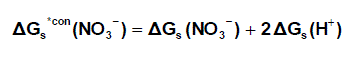(6)

Where ΔG s*con (Pb2+) , ΔG s*con (NO3-) and ΔGs(H+) From the mean values of proton solvation free energies in water and other solvents in literature [12,35,36] relation between these values and the diameter for each solvent taken from literature , straight line was obtained. From this line the proton solvation free energies in pure water and acetone were obtained and found to be 1523 and 1633.61 KJ/mol, respectivity Multiplying each value by its mole fraction in the mixture and then sum the results. The mixed solvent proton free energies were obtained and their values are given in Table (4). Apply equations (5) and (6) we get the conventional Gibbs free energies for the cation and anion and their values are given also in Table (2). Cation conventional free energy values are negative indicating exothermic character and anion values are positive indicating enxothermic character. Both values increase with increase in the mole fraction of acetone due to more solvation and the sum of them gives the values for the neutral salt.

Replica watches